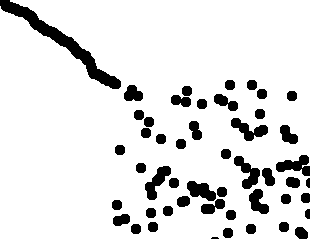CPSC 170 Post Lab 5Visual SortingDue Before Class on Friday, February 12thSometimes it is difficult to figure out how a sorting algorithm works by reading a description. It can be useful to visualize a sorting algorithm to better understand how it works and how it performs in certain situations. You are going to create a program that visualizes sorting algorithms you implemented.

Details

Create a program that displays an array of Integers as circles in a window. Each circle is the same small size but is located at the x coordinate of its index in the array and at the y coordinate of its value. For example, if the Integer at index 42 has a value of 21 then the circle would be drawn at the point (42, 21). The window width is determined by the length of the array and the window height by the range of values in the array. So for example, if the array is of length 500 and the values range between 0 and 400, then the window should be 500 by 400 pixels.

Add to the window a slider with a range equivalent to the number of elements in the Integer array. The position of the slider represents how many elements of the array have been sorted. So for example, if the array size is 500 and the slider is at 50%, then 250 iterations of the sort method should be performed. When the user drags the slider from left to right the array becomes sorted, and when dragging from right to left the array becomes unsorted. Every time the slider is moved, re-sort the same original random array and display the result. This will require storing the original array separately from the sorted array that is drawn to the window.

Add a combo box to the window that can be used to select different sorting algorithms. Lastly, add the bubble sort algorithm to your program. You do not need to add anything extra to this assignment.

Submission: Tar and submit your code on the course blackboard site.